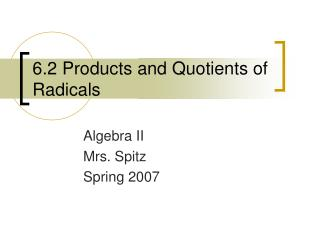# 6.2 Products and Quotients of Radicals - PowerPoint PPT PresentationDownload Presentation6.2 Products and Quotients of Radicals

Download Presentation## 6.2 Products and Quotients of Radicals

- - - - - - - - - - - - - - - - - - - - - - - - - - - E N D - - - - - - - - - - - - - - - - - - - - - - - - - - -
##### Presentation Transcript

1. 6.2 Products and Quotients of Radicals Algebra II Mrs. Spitz Spring 2007

2. Objectives: • Simplify radical expressions using multiplication and division, and • Rationalize the denominator of a fraction containing a radical expression.

3. Assignment • pg. 262 #5-52

4. 2 Methods • Method 1:

5. 2 Methods • Method 2: The result is the same using either method. These examples demonstrate the following principle on the next slide.

6. Product Property of Radicals • For any real numbers a and b, and any integer n, n > 1, • If n is even, then as long as a and b are both nonnegative, and • if n is odd, then When you simplify a square root, first write the prime factorization of the radicand. Then use the product property to isolate the perfect squares. Then simplify each radical.

7. Ex. 1: Simplify If a < 0, then has no real roots, so we must assume that a ≥ 0, we don’t need to write However, b could be negative so we write as |b|.

8. Ex. 2: Simplify

9. Ex. 3: Simplify

10. Ex. 4: Simplify

11. Take a look at the division of radicals to see if there is a quotient property similar to the product property of radicals. What do you think we’ll discover?

12. Take a look at the division of radicals to see if there is a quotient property similar to the product property of radicals. What do you think we’ll discover?

13. Quotient Property of Radicals For any real numbers, a and b, b ≠ 0, and any integer n, n > 1, if all roots are defined.

14. Ex. 5A: Simplify

15. Ex. 5b: Simplify

16. A radical expression is simplified when the following conditions are met: • The index, n, is as small as possible. • The radicand contains no factor (other than one) which is the nth power of an integer or polynomial. • The radicand contains no fractions. • No radicals appear in the denominator.

17. Ex. 6: Simplify

18. Reminder: • Friday, Jan. 19th is MAPS testing for 2nd and 6th periods. You must be here, or it will take you longer to make it up. Since I am giving you a grade (activity) for MAPS testing; I expect you to be there. Deficiencies come up very soon this quarter, and not testing will drop your grade 10%.